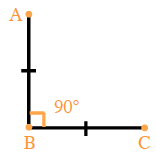### Home > CCG > Chapter 3 > Lesson 3.2.2 > Problem3-70

3-70.

Sketch each triangle if possible. If not possible, explain why not. Homework Help ✎

1. Right isosceles triangles

In order to have a right isosceles triangle, one of the angles must be $90º$ and two of the sides must be the same length. Is it possible to form a triangle with the two requirements? See image below.1. Right obtuse triangles

In order to have a right obtuse triangle, one of the angles must be $90º$and the other greater than $90º$.
What is the sum of the angles in any triangle?
Is this triangle possible?

1. Scalene equilateral triangles

Review the definitions for scalene and equilateral triangles. Does it seem like one triangle could be both?

Since an equilateral triangle has three sides of equal length and a scalene triangle has three sides of different length, an equilateral scalene triangle is impossible.

1. Acute scalene triangles

An acute scalene triangle would have no equal sides and no angles greater than $90º$.

An acute scalene triangle is possible.